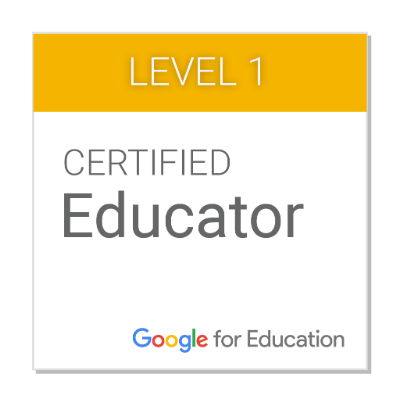Katie Robertson
(423) 623-3811 ext. 319

#### Courses Taught:Bio
I went to NGS K-8 and CCHS 9-12. I graduated from Carson-Newman University with my Bachelors in Education (K-6). I have been teaching for 9 years. This is my second year teaching at Newport Grammar School. My husband's name is Chris and we have two sons, Shane (age 4) and Theo (age 2).
2020-2021 Class List

In-Person Homeroom

Savannah Ball

Makynzie Cutshaw

Theodore Faust

Noah Gregg

Sophia Johnson

Elle Kickliter

Aubree Watts

Virtual Homeroom

Braedon Bolin

Vivienne Cooper

Elias Elallam

Brantley Holder

Johnny Jones

Wyatt Smith

Announcements

See the attached newsletter below for important dates on our December calendar!

Nov. 30-Dec. 4: Math Standards/Objectives and Essential Questions:

1.OA.C.5 Add and subtract within 20 using strategies such as counting on, counting back, making 10, using fact families and related known facts, and composing/ decomposing numbers with an emphasis on making ten (e.g., 13 - 4 = 13 - 3 - 1 = 10 - 1 = 9 or adding 6 + 7 by creating the known equivalent 6 + 4 + 3 = 10 + 3 = 13).

1.OA.C.6 Fluently add and subtract within 20 using mental strategies. By the end of 1st grade, know from memory all sums up to 10.

EQ: How can you use what you know about doubles to find other sums?

EQ: What strategies can you use to solve addition fact problems?

Dec. 7-11: Math Standards/Objectives and Essential Questions:

1.OA.C.5 Add and subtract within 20 using strategies such as counting on, counting back, making 10, using fact families and related known facts, and composing/ decomposing numbers with an emphasis on making ten (e.g., 13 - 4 = 13 - 3 - 1 = 10 - 1 = 9 or adding 6 + 7 by creating the known equivalent 6 + 4 + 3 = 10 + 3 = 13).

1.OA.C.6 Fluently add and subtract within 20 using mental strategies. By the end of 1st grade, know from memory all sums up to 10.

EQ: How can you use a ten frame to add 10 and some more?

EQ: How do you use the make a ten strategy to add?

Dec. 14-18: Math Standards/Objectives and Essential Questions:

1.OA.C.5 Add and subtract within 20 using strategies such as counting on, counting back, making 10, using fact families and related known facts, and composing/ decomposing numbers with an emphasis on making ten (e.g., 13 - 4 = 13 - 3 - 1 = 10 - 1 = 9 or adding 6 + 7 by creating the known equivalent 6 + 4 + 3 = 10 + 3 = 13).

1.OA.C.6 Fluently add and subtract within 20 using mental strategies. By the end of 1st grade, know from memory all sums up to 10.

CC.1.0A.A.2- Solve word problems that call for addition of three whole numbers whose sum is less than or equal to 20, e.g. by using objects, drawings, and equations with a symbol for the unknown number to represent the problem.

CC.1.0A.B.3 Apply properties of operations as strategies to add.

CC.1.0A.C.5 Relate counting to addition (e.g. by counting on to add 2).

EQ: How do you solve addition word problems by drawing a picture?

EQ: How do you solve addition problems?